Electron. J. Differential Equations, Vol. 2018 (2018), No. 02, pp. 1-15.

### Global interval bifurcation and convex solutions for the Monge-Ampere equations Wenguo Shen

Abstract:
In this article, we establish the global bifurcation result from the trivial solutions axis or from infinity for the Monge-Ampere equations with non-differentiable nonlinearity. By applying the above result, we shall determine the interval of, in which there exist radial solutions for the following Monge-Amp\`ere equation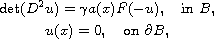where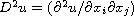is the Hessian matrix of u, where B is the unit open ball of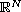,is a positive parameter.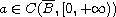is a radially symmetric weighted function and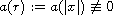on any subinterval of [0, 1] and the nonlinear term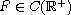but is not necessarily differentiable at the origin and infinity. We use global interval bifurcation techniques to prove our main results.

Submitted June 14, 2017. Published January 2, 2018.
Math Subject Classifications: 34B15, 34C10, 34C23.
Key Words: Global bifurcation; interval bifurcation; convex solutions; Monge-Ampere equations.

Show me the PDF file (295 KB), TEX file for this article.Wenguo Shen Department of Basic Courses Lanzhou Institute of Technology Lanzhou 730050, China email: shenwg369@163.com